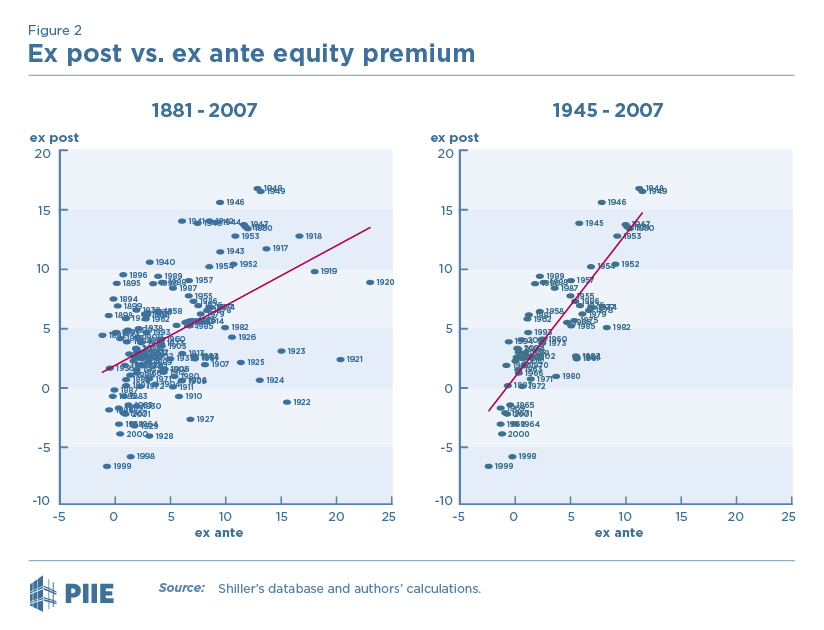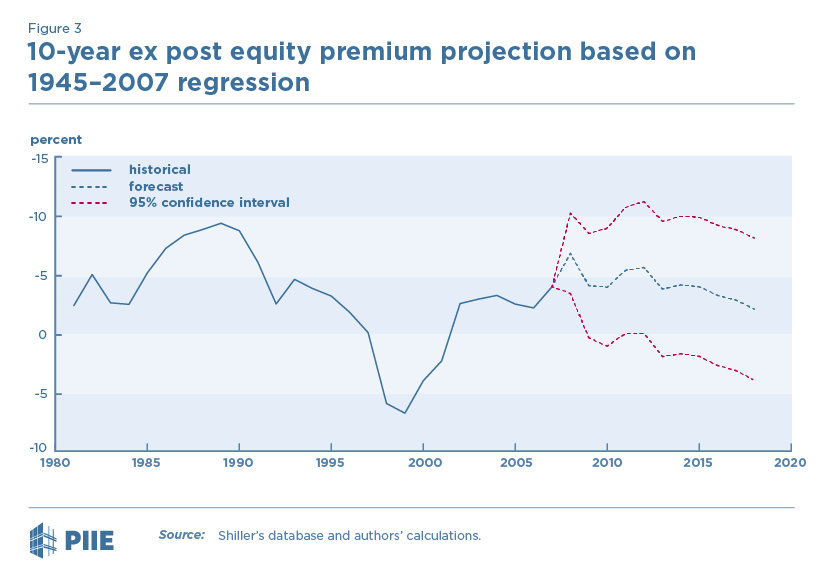# The Stock Market Correction in Context: Are Equities Cheap or Expensive?

November 5, 2018 1:30 PM

October was a bad month for the US stock market. The S&P 500 index fell nearly 7 percent in the month, erasing most of its year-to-date gains. This large swing in the market has left many wondering where we stand; are equities cheap or expensive? The analysis here shows that stocks are expensive relative to their historical average, but they are still likely to outperform bonds over the next 10 years.

One commonly used measure for the valuation of stocks is Robert Shiller’s cyclically adjusted price to earnings ratio (CAPE). The CAPE is calculated as the S&P 500 equity price index divided by the 10-year average of inflation-adjusted earnings per share. The 10-year average is used to smooth out short-term earnings fluctuations related to the business cycle.

At the end of October, the CAPE was 31, which is higher than its historical average, but lower than the dot-com boom of the late 1990s. (See figure 1.) One factor contributing to the high value of the CAPE is the Great Recession of 2008-09, which depressed earnings by more than any time since the 1930s. As 2008 and 2009 drop out of the 10-year average earnings, the CAPE is likely to fall. Figure 1 shows that a CAPE based on 8-year average earnings (thus excluding 2008 and 2009) would be noticeably lower, but still above its historical average.However, any assessment of stock prices must take into consideration the alternative investments available. The primary alternative investment is bonds. Because stocks are riskier than bonds, they normally provide a higher rate of return. The excess return to holding stocks relative to bonds is called the equity premium (EP). An EP calculated using returns for a period in the past is called an ex post EP, whereas an EP calculated using expected returns for a period in the future is called an ex ante EP. The question is whether the ex ante EP is a good predictor of the future ex post EP – that is, does the expected return of stocks in excess of bonds help predict the actual return to stocks in excess of bonds?

As in a previous blog post, the ex ante EP is constructed as the cyclically adjusted earnings-price ratio (the reciprocal of the CAPE) minus the inflation-adjusted yield on the 10-year Treasury note. The ex post EP is the nominal rate of return to holding the S&P 500 index and reinvesting all dividends for 10 years minus the yield on the 10-year Treasury note at the start of the holding period.

Figure 2 shows the relationship between the ex post and ex ante EP over two different periods: 1881 to present and 1945 to present. Each point refers to returns calculated over the same holding period. For example, the ex ante EP in 2000 is based on the earnings-price ratio in 2000, whereas the ex post EP in 2000 is based on actual earnings and stock prices over the following 10 years. The plot suggests a positive relationship between the ex post EP and the ex ante EP, which is stronger for the more recent sample period. As shown in Table 1, regressions confirm that the ex ante EP is a highly significant predictor of the ex post EP. A 1 percentage point increase in the ex ante EP is associated with a 0.4 percentage point increase in the ex post EP over the full sample period, and a 0.9 percentage point increase in the post-war period.Table 1 Regression of ex post equity premium
(1)
1881–2007
(2)
1945–2007
Ex Ante 0.443**
(3.21)
0.927***
(4.71)
Constant 2.324*
(2.44)
0.593
(0.45)
ARMA Residuals
AR(1)
0.892***
(22.41)
0.826***
(9.15)
MA(10) -0.521***
(-4.11)
-0.395**
(-2.78)
R2 0.233 0.658

Note: t statistics in parentheses. * p < 0.05, ** p < 0.01, *** p < 0.001. AR(1) denotes a first-order autoregressive residual and MA(10) denotes a tenth-order moving average error. This specification proved to be a parsimonious way to allow for the MA(1–9) errors induced by the construction of ex post EP as a 10-year moving average of returns, but the results are not sensitive to using MA(1–9) errors.

Figure 3 shows the predicted values and confidence intervals for the ex post EP in 2008 through 2018 based on the post-1945 regression shown in Table 1. The predictions start in 2008 because they require 10 years of future earnings data, so the last observed ex post EP was in 2007. The prediction for yearend 2018 is based on the October 31 level of the ex ante EP, 1.7 percent, which is at the low end (18th percentile) of its distribution since 1945. Stock prices are indeed expensive in historical context. Nevertheless, stocks are likely to outperform bonds over the next 10 years; the model implies about a 25 percent chance that stocks perform worse than bonds. Moreover, if earnings in 2018 and 2019 come in close to their levels after 2009 rather than their levels in 2008 and 2009, and if stock prices and bond yields remain at their October 31 level through the end of 2019, then the ex ante EP would rise to 2.1 and the model would suggest only a 20 percent probability that stocks would perform worse than bonds over the following 10 years.## Notes

1. Because the 8-year CAPE does not include any recession at all, a better CAPE estimate might lie between the 8-year and 10-year CAPEs.

2. The earnings-price ratio is effectively an inflation-adjusted rate of return on stocks because the value of corporate assets, and hence both future earnings and the equity price, increases with inflation. The Treasury yield is adjusted by subtracting a 10-year average of past inflation.

3. This conclusion assumes that the ex post EP is normally distributed. If asset returns have fatter tails than a normal distribution, as is widely believed, then the probability of underperformance is somewhat greater than 25 percent.

## More on This Topic

Policy Brief

November 2015
Policy Brief

November 2014
PIIE Briefing

### The US-China-Europe Economic Reform Agenda

Nicholas Borst (Federal Reserve Bank of San Francisco), Nicholas R. Lardy (PIIE), Cullen S. Hendrix (PIIE), Marcus Noland (PIIE), Ryan Rutkowski (PIIE), Arvind Subramanian (PIIE) and Nicolas Véron (PIIE)

May 2014